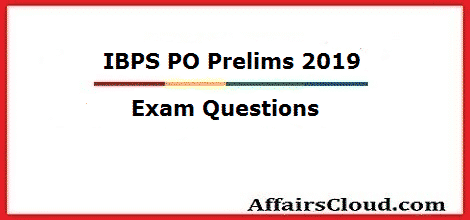#Questions asked in IBPS PO Prelims Exam 2019 – October 19

Questions asked in IBPS PO Prelims Exam October 19, 2019, are listed below. IBPS PO Prelims Exam 2019 has three sections namely Quantitative Aptitude, Reasoning Ability, and English. The overall exam is said to be moderate. If you have any more questions from the exam, then kindly share it in the comment section.#### Questions asked in IBPS PO Prelims Exam 2019 – October 19

Quant:

Approximation:

1. 18.122 × 2.01 + 30.092
2. 580.11 + 720.12 – ? = 24.992
3. √1300 + 5.99 × 3.05
4. 80.18 % of 499 – 6.001% of 80
5. 724.95 ÷ 24.99 + 64 ÷ 7
6. 29.98% of 200.16+71÷5

1. 20x2 + 9x + 1 = 0
2y2 + 3 y + 1 = 0
2. x2 = 81
y2-12y+27=0
3. x2-18x+80=0
y2-17y+72=0
4. x2+7x+12=0
y2+5y+6=0
5. x2=16
y2+27=31
6. 12x2+7x+1=0
15y2+11y+2=0

Arithmetic:

1. The perimeter of a rectangle is 4 cm less than the circumference of the circle. If the breadth of the rectangle is equal to the radius of the circle and also the breadth is half of the length of the rectangle, what is the area of the rectangle in sq. cm?

**Questions are collected purely based on the feedback from aspirants. If you find any error then kindly report it.

Click Here To View IBPS PO Prelims 2019 Exam Review –  October 19

Click Here To View IBPS PO Prelims 2019 Exam Review –  October 12